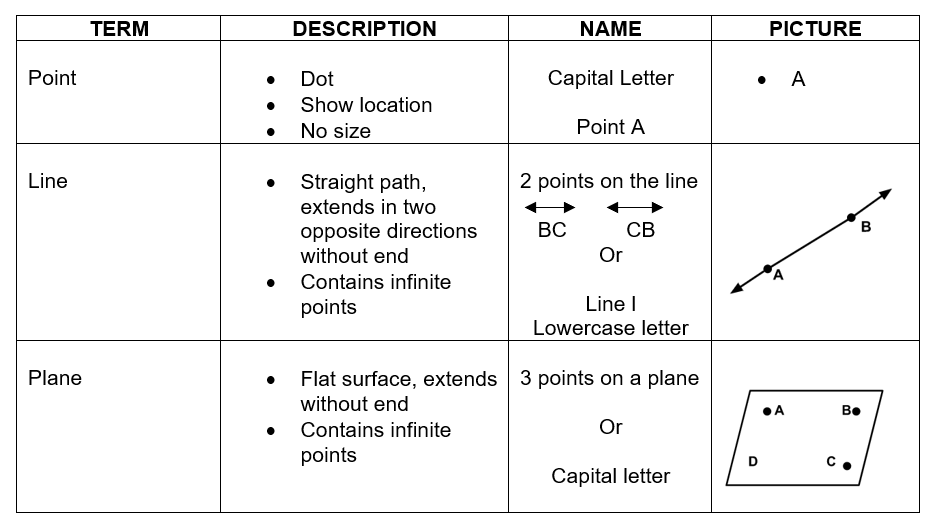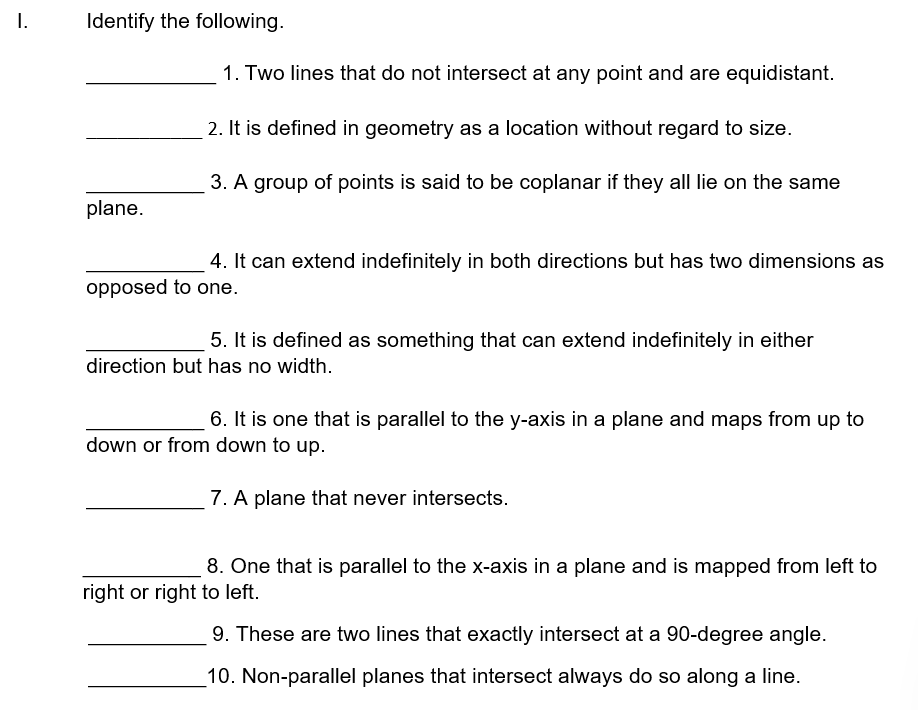# Worksheet 1.1 Points, Lines, and Planes (with answer key)

There are several undefined terms in geometry, including point, line, and plane. All terms in geometry can be defined based on these three ambiguous terms. A point is defined in geometry as a location without regard to size. In contrast to a plane, which can extend indefinitely in both directions but has two dimensions as opposed to one, a line is defined as something that can extend indefinitely in either direction but has no width.

What are the “Worksheet 1.1 Points, Lines, and Planes (answer key)”?

This worksheet will explore the concept of points, lines, and planes. It is important to understand geometric figures. With the help of points, we can label and identify geometric figures and with the help of lines, we can draw the figures, and a plane is a flat, two-dimensional surface that extends indefinitely.

Regarding the specific subject, this worksheet offers in-depth details. Students can better understand the idea of Points, Lines, and Planes in geometry by working through the worksheet provided.

Instructions on how to use the “Worksheet 1.1 Points, Lines, and Planes (answer key).”

Try to provide answers to the following exercises after studying the concept and providing examples.

## Conclusion

This worksheet provides information for students with full details with it. Words that do not have formal definitions but there is an agreement about what they mean.

If you have any inquiries or feedback, please let us know.

## Worksheet 1.1 Points, Lines, and Planes (with answer key)Types of points

The geometry contains various kinds of points. Let’s discuss them so we can quickly recognize them.

1. Non-Collinear and Collinear Points

Are those that lie along a single straight line with three or more other points. Non-collinear points are a group of points that do not all lie along the same line.

1. Points that are Coplanar and Non-Coplanar

A group of points is said to be coplanar if they all lie on the same plane. Non-coplanar points are a group of points that do not share a plane.

Types of Lines

Similar to different types of points, different types of lines also exist, and they can be easily distinguished from one another based on their distinctive characteristics.

1. A horizontal line – is one that is parallel to the x-axis in a plane and is mapped from left to right or right to left.
1. A vertical line – is one that is parallel to the y-axis in a plane and maps from up to down or from down to up.
1. Intersecting lines- are two lines that cross each other and come together at a single point. The intersection is the place where they come together.
1. Perpendicular Lines – are two lines that exactly intersect at a 90-degree angle.
1. Parallel Lines – Two lines are said to be parallel if they do not intersect at any point and are equidistant.

Types of Planes

1. Parallel Planes – Parallel planes are planes that never intersect.
1. Intersecting Planes – Non-parallel planes that intersect always do so along a line and are said to be intersecting planes. There can only be one line where two planes can intersect.

## Worksheet